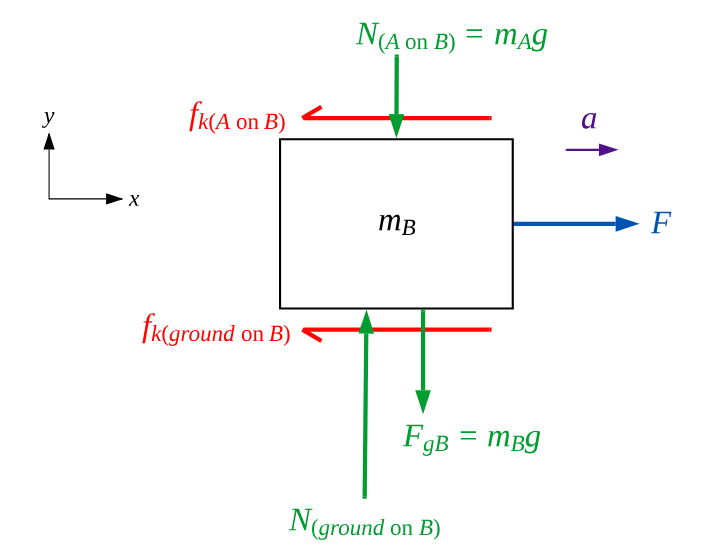# Problem: The 1.0 kg block in the figure below is tied to the wall with a rope. It sits on top of the 2.0 kg block. The lower block is pulled to the right with a tension force of 40 N. The coefficient of kinetic friction at both the lower and upper surfaces of the 2.0 kg block is k = 0.35.What is the acceleration of the 2.0 kg block?______m/s2

###### FREE Expert Solution

Frictional force:

$\overline{){\mathbf{f}}{\mathbf{=}}{\mathbf{\mu }}{\mathbf{N}}}$

FBD for the bottom block, calling the top block A and the bottom block B:ΣF = T fk(A on B) - fk(ground on B) = ma

92% (173 ratings)###### Problem Details

The 1.0 kg block in the figure below is tied to the wall with a rope. It sits on top of the 2.0 kg block. The lower block is pulled to the right with a tension force of 40 N. The coefficient of kinetic friction at both the lower and upper surfaces of the 2.0 kg block is k = 0.35.

What is the acceleration of the 2.0 kg block?
______m/s2